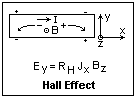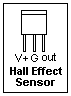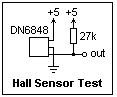## The Hall Effect and Hall Effect Sensors

Hall effect sensors can solve many problems, so it is useful to know about them. Also, the Hall Effect itself is worth understanding, so we'll talk about that as well. What we are sensing is magnetic field B, which in MKS units is measured in Tesla, which hardly anyone uses. A better unit is the gauss, and 10,000 G are one T. Many small permanent magnets can scratch up a few hundred gauss in their vicinity, while the earth's field is in the region of one gauss. Magnetic materials (iron) may saturate at some 15,000 G. This should orient you as to orders of magnitude.

The force on a point charge q is F = qE + qv x B. This is a vector equation, giving direction as well as magnitude. q is the charge in coulomb, v is the velocity in m/s, E is the electric field in V/m, and B the magnetic field in T. We can do quite a lot with this equation, but strictly it applies only to a free electron in space, not to the crowd of electrons in a metal or semiconductor. Many simple relations can be obtained, rather illegally, with its use that can be verifed by better calculations. On the other hand, there are many questions that the independent electron model cannot answer correctly, or at all.The Hall Effect has to do with a current I flowing in a conductor, say in the x-direction, in a region with a magnetic field B in the z-direction. An electric field E, called the Hall field, appears in the conductor in the y direction. These relations are shown in the diagram on the right. The + and - represent charge carriers of positive and negative charge, respectively, carrying current in the direction of I. Each is driven towards the lower edge of the conductor by the magnetic force at right angles to its velocity. To keep them moving straight, charges are necessary to establish an electric field in the y-direction to counterbalance the magnetic force, and these charges are shown by the small + and - symbols. Note that the direction of the field is different for different charges--positive for + charges, and negative for - charges. These relations work for any directions, not just the perpendicular ones considered here. However, this only introduces cosines and sines which complicate matters without adding anything new.

The Hall coefficient RH is the factor multiplying the product of the current density and the magnetic field to get the Hall field. We can derive a useful expression by equating the magnetic and electric forces: qvB = qE. Multiplying and dividing the left-hand side by nA, where n is the number of charge carriers per unit volume and A the cross-sectional area of the conductor, and identifying I = nAqv, we find IB/nA = qE, or E = (1/nq)(I/A)B = (1/nq)JB, so that RH = 1/nq. The Hall coefficient is just the reciprocal of the total current-carrying charge in the conductor, and has the same sign as the sign of this charge. For most metals, the Hall coefficient is negative, as expected if the charge carriers are electrons. In beryllium, cadmium and tungsten, however, the coefficient is positive. In these metals, the charge carriers are holes, which act like positive charges. In a semiconductor, the Hall coefficient can be positive or negative, depending on whether it is P or N type. An intrinsic semiconductor, with equal numbers of mobile electrons and holes, is predicted to have a zero Hall coefficient.Several manufacturers make Hall Effect sensors in which a sensitive comparator detects the Hall voltage and provides a logic output. Considerable Schmitt trigger action is provided, so that the transition is sharp and does not chatter. In the DN6848 sensor, the H-L transition occurs at about 225 gauss, while the L-H transition occurs at about 5 gauss. The sensor is sensitive to only one direction of the magnetic field. Other sensors require a reversal of the magnetic field, from about 175 gauss in one direction to 175 gauss in the other. The output may have an internal pullup resistor, or may be open-collector. The DN6848 is open-collector, and a 27k pullup resistor is suggested. The power supply is 4.5 to 16 V, and may be different from the logic voltage pulling up the output. The pinout of the DN6848 is shown at the right; it is sensitive to a magnetic flux out of the page (south pole in front).A circuit for testing the DN6848 is shown at the left. The output swings from +5 to ground. It will detect the presence of a south pole near to its front without physical contact, which is its great advantage. Using it as a tachometer would be a good test, but the mechanical arrangements would take some care and effort. Static switching would be easier to arrange, as detecting the opening and closing of a door or drawer. Magnets are available from Edmund Scientific and some surplus houses.

The Hall Effect was discovered in 1879 by E. H. Hall (not the more famous C. M. Hall of electrolytic aluminum). At that time, the electron was unknown, and while many such interesting effects were observed, by workers like Hall and Edison, they could not be correlated or understood. People really didn't know what electricity was at that time. Now we know, and things are much clearer and more interesting. The Hall effect is important in semiconductor physics because it gives the carrier density and charge directly.

There are other ways to sense magnetic fields, and some of them may be included here later.